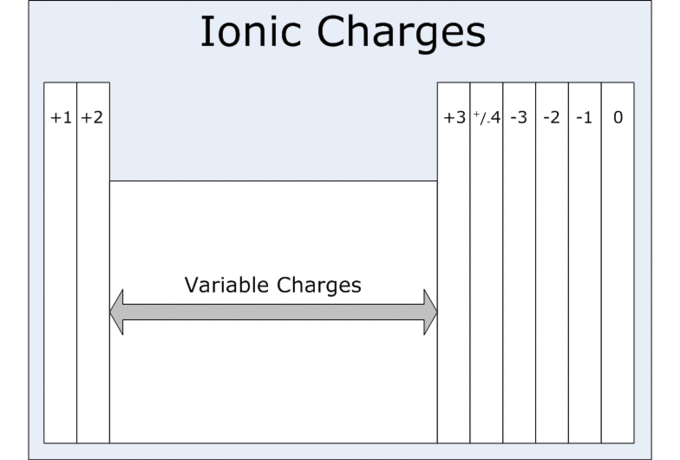## Oxidation States

#### Learning Objective

• Predict the oxidation states of common elements by their group number.

#### Key Points

• The oxidation state of a pure element is always zero.
• The oxidation state for a pure ion is equivalent to its ionic charge.
• In general, hydrogen has an oxidation state of +1, while oxygen has an oxidation state of -2.
• The sum of the oxidation states for all atoms of a neutral molecule must add up to zero.

#### Terms

• reductionthe gain of electrons, which causes a decrease in oxidation state
• oxidationthe loss of electrons, which causes an increase in oxidation state

Oxidation state indicates the degree of oxidation for an atom in a chemical compound; it is the hypothetical charge that an atom would have if all bonds to atoms of different elements were completely ionic. Oxidation states are typically represented by integers, which can be positive, negative, or zero. In some cases, the average oxidation state of an element is a fraction, such as 8/3 for iron in magnetite (Fe3O4).

The highest known oxidation state is +8 in the tetroxides of ruthenium, xenon, osmium, iridium, hassium, and some complexes involving plutonium; the lowest known oxidation state is −4 for some elements in the carbon group.

An atom’s increase in oxidation state through a chemical reaction is called oxidation, and it involves a loss of electrons; an decrease in an atom’s oxidation state is called reduction, and it involves the gain of electrons.

## General Rules Regarding Oxidation States

1. The oxidation state of a free element (uncombined element) is zero.
2. For a simple (monoatomic) ion, the oxidation state is equal to the net charge on the ion. For example, Cl has an oxidation state of -1.
3. When present in most compounds, hydrogen has an oxidation state of +1 and oxygen an oxidation state of −2. The exceptions to this are that hydrogen has an oxidation state of −1 in hydrides of active metals (such as LiH), and an oxidation state of −1 in peroxides (such as H2O2) or -1/2 in superoxides (such as KO).
4. The algebraic sum of oxidation states for all atoms in a neutral molecule must be zero. In ions, the algebraic sum of the oxidation states of the constituent atoms must be equal to the charge on the ion.

## Predicting Oxidation States

Generally, the oxidation state for most common elements can be determined from their group number on the periodic table. This is summarized in the following chart:Typical oxidation states of the most common elements by groupTransition metals are not included, as they tend to exhibit a variety of oxidation states.

The above table can be used to conclude that boron (a Group III element) will typically have an oxidation state of +3, and nitrogen (a group V element) an oxidation state of -3. Keep in mind that oxidation states can change, and this prediction method should only be used as a general guideline; for example, transition metals do not adhere to any fixed rules and tend to exhibit a wide range of oxidation states.

As stated in rule number four above, the sum of the oxidation states for all atoms in a molecule or polyatomic ion is equal to the charge of the molecule or ion. This helps determine the oxidation state of any one element in a given molecule or ion, assuming that we know the common oxidation states of all of the other elements. For example, in a sulfite ion (SO32-), the total charge of the ion is 2-, and each oxygen is assumed to be in its usual oxidation state of -2. Because there are three oxygen atoms in sulfite, oxygen contributes $3\times-2=-6$ to the total charge. Therefore, sulfur must have an oxidation state of +4 for the overall charge on sulfite to be 2-: $(+4-6=-2).$

Do not confuse the formal charge on an atom with its formal oxidation state, as these may be different (and often are different, in polyatomic ions). For example, the charge on the nitrogen atom in ammonium ion NH4+ is 1+, but the formal oxidation state is -3—the same as it is for nitrogen in ammonia. In the case between ammonium and ammonia, the formal charge on the N atom changes, but its oxidation state does not.